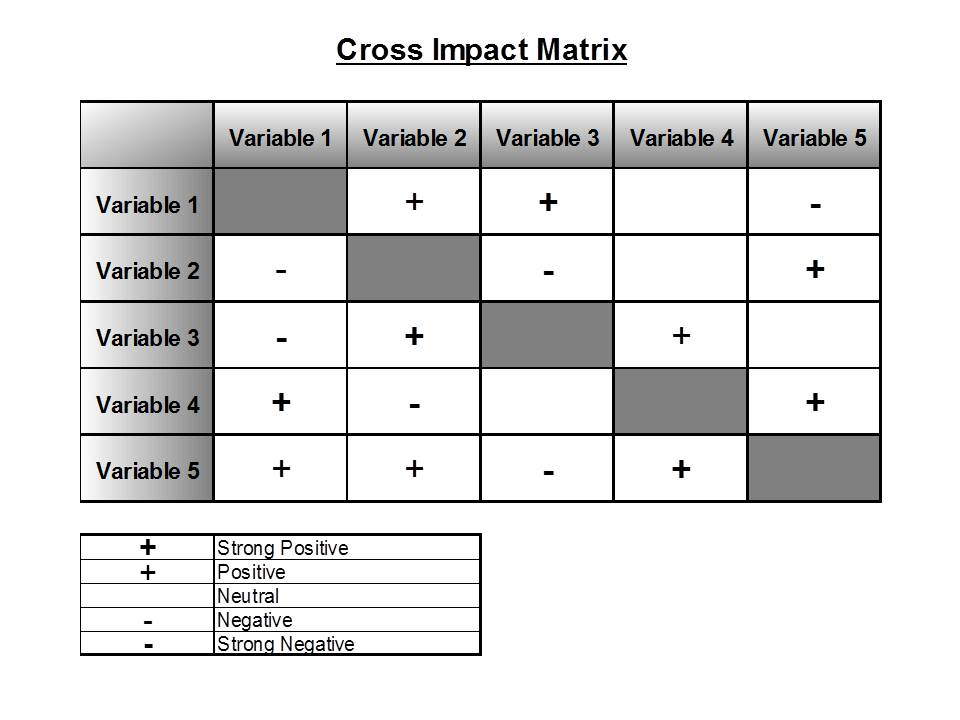Not a member yet?# Cross Impact Matrix

### Determine how the relationships between variables impact future events.Cross Impact Matrix tool was developed to answer a simple question:

“Can forecasting be based on perceptions about how future events may interact?”.

This method helps you examine how variables influence future events. It may also show how much each variable influences the outcome.

Create a Cross Impact Matrix

Step 1:  Begin creating your Cross Impact Matrix by defining a list of variables. The variables are actions or events that have some effect on your issue when they are changed. Look for relevant variables in Forces and Chronology. Any variable not included will obviously have no influence. Including variables that are not pertinent will only complicate the analysis.

Step 2:  Create a matrix (see our example of a Cross Impact Matrix diagram below).  List the variables down the left-hand column of the matrix. Also list the same variables across the top.

Step 3:  Start with the variable at the top of the left-hand column. Compare it to each variable going across the matrix from left-to-right. For each pair of variables, ask the question, “How much does a change in the first variable affect the second variable?”. In the box representing the paired comparison, write down the probability of how much one variable influences the other.

Step 4:  Repeat this process, filling-in each box of the matrix representing a paired comparison. The probabilities can be determined though mathematics or by a simple plus or minus system. One popular method is to use a bold plus (+) for a “strong positive” influence, plus (+) for “positive”, blank for “neutral”, minus (-) for "negative", and bold minus (-) for "strong negative".

It may be helpful to record your thoughts, about the interaction between each pair of variables, as you're doing this exercise for future reference.

Step 5:  Analyze your Cross Impact Matrix:

• Determine the cause and effect relationships.
• Look for significant interactions between your variables. Also, look at combining some of your variables (that seem to be related to each other) and see how they interact with other variables.
• Determine the causal linkages between all of your variables and how they interact as a whole system (see our Causal Flow Chart and Cause and Effect Fishbone Diagram tools).
• Examine all assumptions about the relationships between your variables (see our Assumptions tool).

Cross Impact Matrix Form: Use this form to see how your variables influence future outcomes.